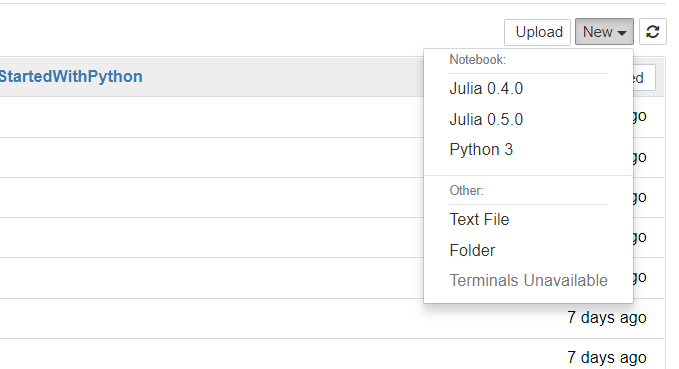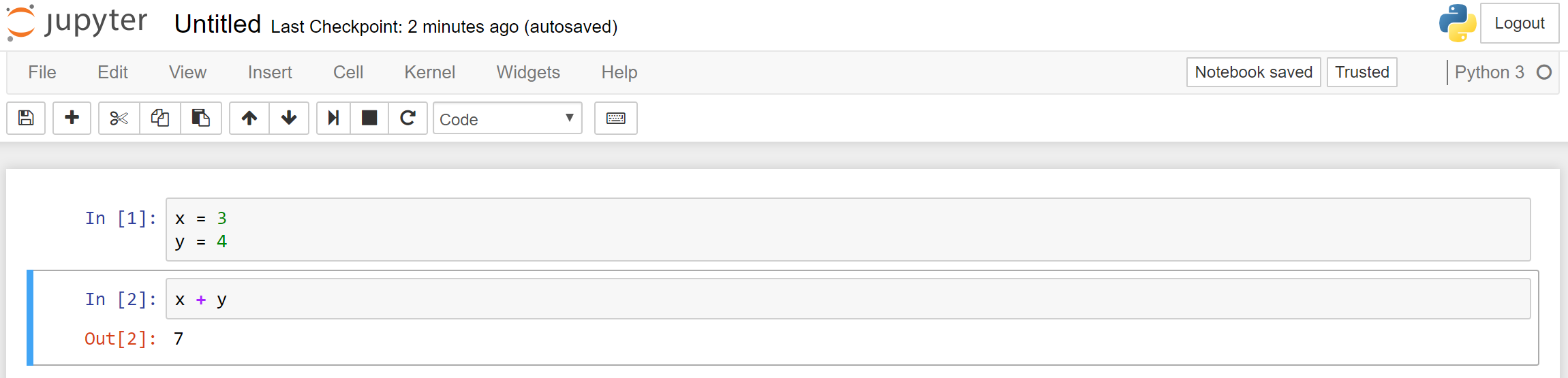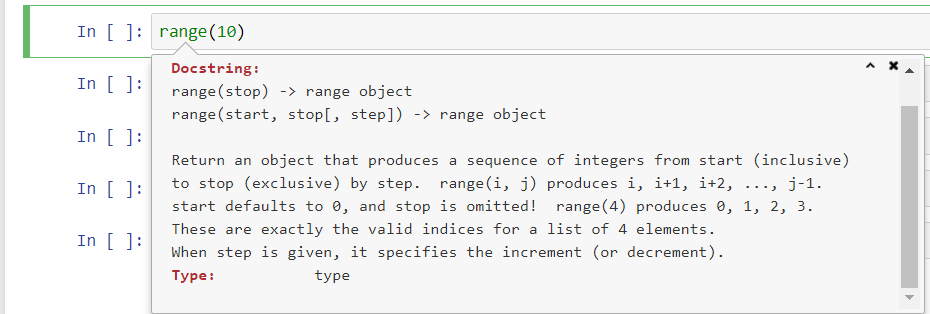# Jupyter记事本¶

Jupyter记事本类似于IPython命令行，但，相比起IPython每次编写运行一行代码的形式，你可以轻松地编辑和重新执行任何在记事本中的代码。同时，你可以保存一个记事本，然后在未来去运行和修改它。Jupyter记事本提供很多特别实用的功能。比如说，你可以在记事本中嵌入可视化的多媒体（如表格和图画）并利用Markdown语法编写方便美观的文本来演示和解释记事本的内容。

## JupyterLab¶

JupyterLab是由Project Jupyter团队开发的一个全新的网页端交互界面。通过它，你可以在你的浏览器中编辑并运行Jupyter记事本，命令行，和文本编辑器。除了各种功能和相比Jupyter记事本更加成熟的用户界面之外，JupyterLab还提供了可以用来显示数据，图片，和代码输出的在Jupyter记事本之外的可移动窗口。这将允许你高效地进行数据科学工作。

## 运行记事本服务器并创建记事本¶Jupyter记事本并不使用互联网

## 记事本小节¶

x = 3
y = 4


x + y## 样例记事本¶

:

import numpy as np
import matplotlib.pyplot as plt

# 这会告诉Jupyter将matplotlib的图表嵌入在记事本中
%matplotlib notebook

:

def sinc(x):
return np.sin(x) / x

def d_sinc(x):
"sinc函数的导数"
return np.cos(x)/x - np.sin(x)/x**2

:

# 用1000个平均分布在[-15, 15]之间的点求值
x = np.linspace(-15, 15, 1000)
f = sinc(x)
df = d_sinc(x)

:

# 绘制sinc函数和它的导数
fig, ax = plt.subplots()
ax.plot(x, f, color="red", label=r"$sinc(x)$")
ax.plot(x, df, color="blue", ls="--", label=r"$\frac{d(sinc(x))}{dx}$")

ax.set_title("Example Notebook Plot")
ax.set_xlabel(r"$x$ [radians]")

ax.grid(True)
ax.legend();


## 熟悉Jupyter记事本¶

• 在当前小节上方创建一个新的小节：a

• 在当前小节下方创建一个新的小节：b

• 删除当前小节：dd

• 重启记事本壳（杀死所有进程并删除所有定义的变量）：00

• 将当前小节的类型改为“代码”：y

• 将当前小节的类型改为“Markdown”：m

• 执行当前小节：<CTRL>+<ENTER>

• 执行当前小节并在下方创建新小节：<SHIFT>+<ENTER>### 将Jupyter记事本用于其它语言¶

Jupyter记事本不只支持Python。你可以在Jupyter中为任何编程语言开发一个“壳”（Kernel）。其实很多重要的编程语言都有着自己相对应的壳：

### Visual Studio Code的Jupyter记事本支持¶

Visual Studio Code在近期开始官方支持Jupyter记事本。这意味着你可以在Visual Studio Code IDE中直接编辑Jupyter记事本，并可以享受如代码完成，调试，和查看变量的额外功能。